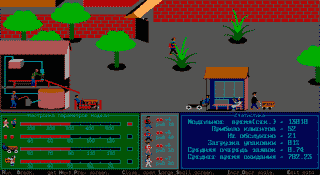# Simulation Systems Department.

 117967 Vavilova 40, Moscow. Tel: +7 (095) 135-62-04, Fax: +7 (095) 135-61-59. In Russian E-mail: simul@ccas.ruThe department of the Computing Center of Russian Academy of Science "Simulation Systems" had been foundated by initiative of Academician N.N. Moiseev in 1973. In the department the methods and means of mathematical simulation of complicated processes, system, phenomena are developed. Main aim of the activity is promotion of mathematical modeling into new complicated area of studies and applications. The main themes of research are:

Methodological aspect of simulation. Development of technology, which unify mathematical and humanitarian methods of analysis and prognosis of complicated processes (systems, phenomena).

Development of mathematical models of concrete complicated processes, development of corresponding computer simulation systems, investigation the processes by means of simulation experiments and simulation games.

Development of instrumental means of mathematical simulation.

Development of decomposition theory of mathematical models (mathematical objects).

Reduction of nonlinear dynamic cintrol systems.

Investigation of complicated control systems by optimization and game-theoretical models.

Selected articles by members of the Department (in Russian)